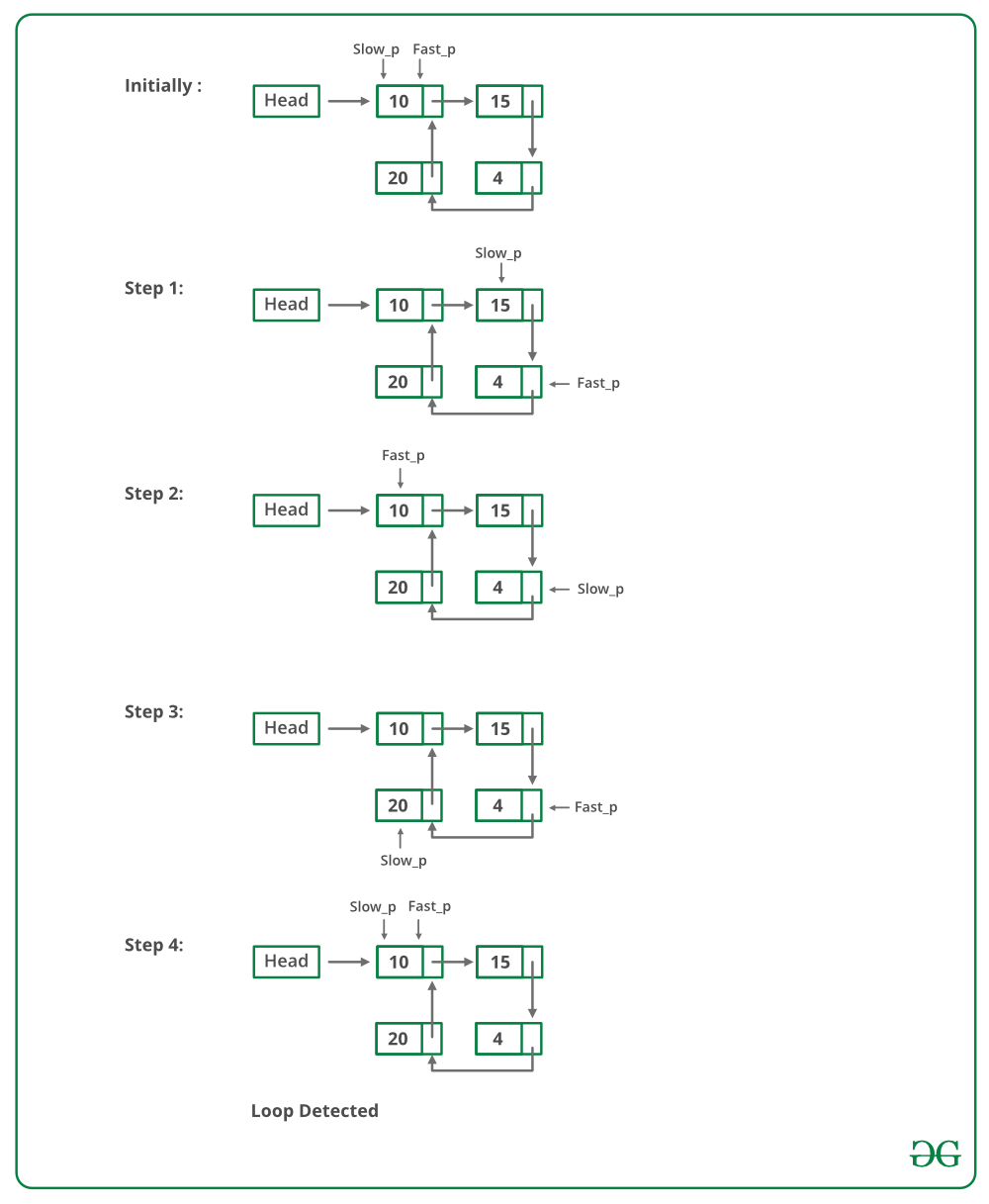Detect loop in a linked list

• Difficulty Level : Easy
• Last Updated : 19 Jan, 2022

Given a linked list, check if the linked list has loop or not. Below diagram shows a linked list with a loop.The following are different ways of doing this.

Solution 1: Hashing Approach:

Traverse the list one by one and keep putting the node addresses in a Hash Table. At any point, if NULL is reached then return false, and if the next of the current nodes points to any of the previously stored nodes in  Hash then return true.

Javascript



Output

Loop found

Complexity Analysis:

• Time complexity: O(n).
Only one traversal of the loop is needed.
• Auxiliary Space: O(n).
n is the space required to store the value in hashmap.

Solution 2: This problem can be solved without hashmap by modifying the linked list data structure.
Approach: This solution requires modifications to the basic linked list data structure.

• Have a visited flag with each node.
• Traverse the linked list and keep marking visited nodes.
• If you see a visited node again then there is a loop. This solution works in O(n) but requires additional information with each node.
• A variation of this solution that doesn’t require modification to basic data structure can be implemented using a hash, just store the addresses of visited nodes in a hash and if you see an address that already exists in hash then there is a loop.

Javascript



Output

Loop found

Complexity Analysis:

• Time complexity:O(n).
Only one traversal of the loop is needed.
• Auxiliary Space:O(1).
No extra space is needed.

Solution 3: Floyd’s Cycle-Finding Algorithm
Approach: This is the fastest method and has been described below:

• Traverse linked list using two pointers.
• Move one pointer(slow_p) by one and another pointer(fast_p) by two.
• If these pointers meet at the same node then there is a loop. If pointers do not meet then linked list doesn’t have a loop.

The below image shows how the detectloop function works in the code:Implementation of Floyd’s Cycle-Finding Algorithm:

Javascript



Output

Loop found

Complexity Analysis:

• Time complexity: O(n).
Only one traversal of the loop is needed.
• Auxiliary Space:O(1).
There is no space required.

How does above algorithm work?
Please See : How does Floyd’s slow and fast pointers approach work?

Solution 4: Marking visited nodes without modifying the linked list data structure
In this method, a temporary node is created. The next pointer of each node that is traversed is made to point to this temporary node. This way we are using the next pointer of a node as a flag to indicate whether the node has been traversed or not. Every node is checked to see if the next is pointing to a temporary node or not. In the case of the first node of the loop, the second time we traverse it this condition will be true, hence we find that loop exists. If we come across a node that points to null then the loop doesn’t exist.

Below is the implementation of the above approach:

Javascript



Output

Loop Found

Complexity Analysis:

• Time complexity: O(n).
Only one traversal of the loop is needed.
• Auxiliary Space: O(1).
There is no space required.

Solution 5: Store length

In this method, two pointers are created, first (always points to head) and last. Each time the last pointer moves we calculate no of nodes in between first and last and check whether the current no of nodes > previous no of nodes, if yes we proceed by moving last pointer else it means we’ve reached the end of the loop, so we return output accordingly.

Javascript



Output

Loop Found

Complexity Analysis:

• Time complexity: O(n2)
• Auxiliary Space: O(1)

Another Approach:

1. This is the simplest approach of the given problem, the only thing we have to do is to assign a new value to each data of node in the linked list which is not in the range given.
2. Example suppose (1 <= Data on Node <= 10^3) then after visiting node assign the data as -1 as it is out of the given range.

Follow the code given below for a better understanding:

Javascript



Output

1

Time Complexity: O(N)

Auxiliary Space: O(1)